You are here : Main projects | The Apollo Gauss cannon | 2. Theory of operation

The Apollo Gauss Cannon
April 2014

# 2.  Theory of operation

A Gauss cannon, or coilgun, is a type of electromagnetic cannon, i.e. a device that fires projectiles using the electromagnetic force. This specific type uses ferromagnetic projectiles that are accelerated using the magnetic field generated by an inductor which is part of (R)LC circuit. We will carefully review all these terms before moving on to the actual theory of operation of a Gauss cannon. After that, we'll analyse the fire sequence properly said a little bit deeper.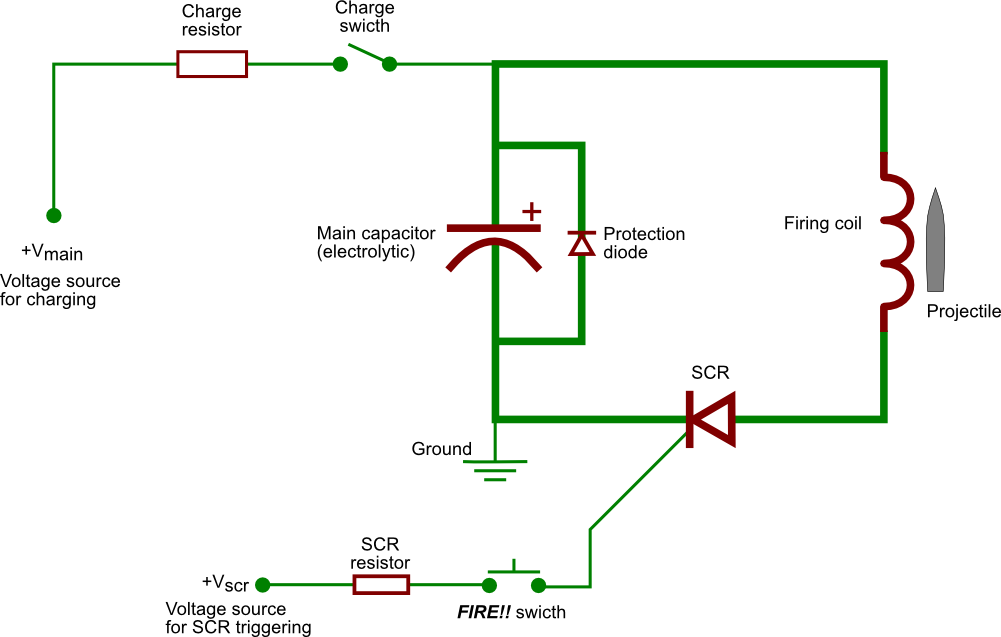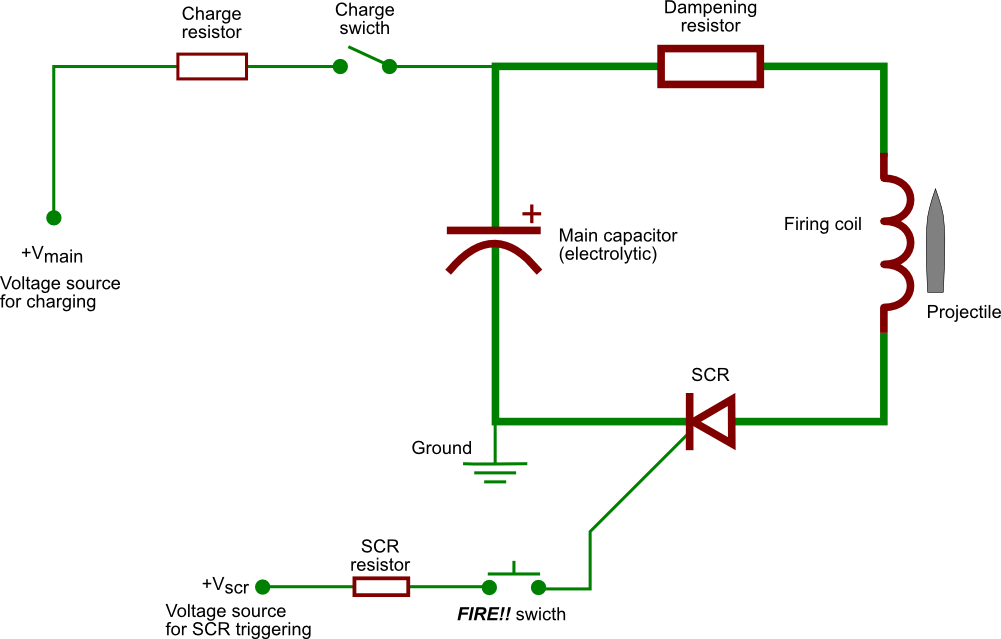Figure 2.1 — Two very basic designs of Gauss cannons. The LC circuit version (top) and the critically-damped RLC circuit version (bottom). The bolder lines indicate the high-current capacity firing circuit itself in each case.

Its name refers to Carl Friedrich Gauss (1777 – 1855), a German mathematician and physicist who made monumental contributions to science. Although he didn't invent what is today referred to as the "Gauss cannon", he helped in laying down the mathematical formulation of electromagnetism, from which all the principles underlying the working of the gun arise.

Note : The first sections, 2.1 and 2.2, are mostly reminders of elementary physics. If you remember your 101 courses well enough, you may want to jump directly to section 2.3, which deals with Gauss cannon theory of operation properly said.

## 2.1   Reminders on magnetism

This section deals with some elementary aspects of magnetic fields and establishes a few useful formulas regarding them. Although the magnetic and the electric fields really are two facets of the same object, it can be convenient to treat them separately, which is why we'll do it here. As mentioned it in the introductory paragraph, a Gauss cannon uses the magnetic field produced in a solenoid (a coil) to accelerate a piece of ferromagnetic material. Let's define these terms precisely before going on any further.

### 2.1.1   Magnetic field in a solenoid

We'll start by quickly calculating the shape of the magnetic field in a solenoid, using Maxwell's equations. Let's consider an infinite solenoid traversed by a current I as shown in the picture below. By infinite, we mean that we'll discard the "edge effects" and focus only on the centre part.Figure 2.2 — Depictions of a real-life solenoid and an idealized one.

Let us first use the symmetries of the system to simplify the problem. The only symmetry here is translational invariance on the z-axis, so that every plan crossing the solenoid perpendicularly is a symmetry plan. From the properties of the magnetic field, we deduce it must be independent of z and directed on the z-axis : B(x,y,z) = B(x,y)uz.

We'll make use of Ampère's theorem all along so let us remind it here. The theorem states that the circulation of the magnetic field B around a certain contour C is proportional to the current I passing through this contour. More precisely,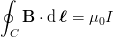Let's first choose a rectangular contour inside the solenoid. As no current passes through it, the contour integral is zero. This tells us the magnetic field is the same at both the x coordinates of the rectangle's horizontal sides, from which we deduce the magnetic field is independent of x inside the solenoid. Doing the same reasoning on the yz-plane tells us the magnetic field is also independent of y inside the solenoid. The magnetic field is therefore constant inside the solenoid : Bin = Binuz. If we now repeat those two steps outside the solenoid, we find that the magnetic field is also constant (as there's still no current crossing the loop we've chosen) : Bout = Boutuz. From this discussion, we conclude that the field has the following form :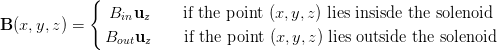We have already greatly simplified the problem at this point. Now all we have to do is find the numerical constants Bin and Bout.

We are now going to determine Bout using a physical argument. We consider that, far away from the solenoid, all the physical fields are vanishing. Therefore, as the magnetic field outisde the solenoid is zero at infinity, and is constant, we conclude that Bout = 0. Note that this argument is actually flawed since the solenoid is here considered to be an infinite object as well, but the result Bout = 0 can be proven rigorously (and certainly is in your Physics 101 course).Now, let's move to Bin. To determine it, we once again use Ampère's theorem, this time on a rectangle crossing the solenoid. The rectangle crosses the solenoid n times, and its horizontal sides have a length d, as shown in the picture on the side. We find that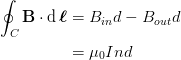Now, as Bout = 0, we find that Bin = μ0nI. We have now completely determined the field generated by our infinite solenoid! Indeed we have found that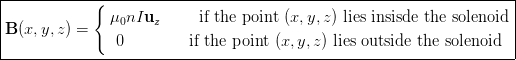(2.1)

where n becomes the number of spires of the coil. Figure 2.3 below depicts the field lines in a realistic (non-infinite) solenoid. We see that the field lines well inside the coil are indeed completely horizontal, as we have shown. The edge effects arising for the spatial finiteness of the solenoid (which we neglected in our quick treatment) are also visible.Figure 2.3 — Realistic depictions of the magnetic field lines generated by a solenoid.
[Image credits: Geek3 for Wikipedia]

### 2.1.2   Magnetism in matter

We already know, through Maxwell's equations, that a current produces a magnetic field, and we just examined what that field looks like in a solenoid, but we haven't talked about the effects of this magnetic field on matter. This section will treat the macroscopic aspects of magnetism in matter, which are essential to understand why is the projectile is attracted with so much force into the cannon's firing coil when current is discharged into it.

The microscopic aspects, i.e. the quantum-mechanical origins of magnetism, won't be discussed here but the interested reader will find a comprehensive summary in the related Wikipedia entry.

#### Magnetic field and magnetisation

A magnetic material will display a magnetisation M (a vectorial quantity) per unit of volume, which is defined as following: dμ = MdV, where dμ represent the magnetic moment per unit volume dV. When a linear and isotropic material (such as the iron rod we're using as projectile) is placed into a external field B, we can definewhere χ is the magnetic susceptibility of the material, and μ0 the vacuum permittivity. This formula establishes the effective link between the magnetic field and magnetisation. Now, by introducing a new quantity μr = χ + 1, the relative permittivity, we can rewrite our previous formula into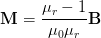(2.2)

One can also define a "rescaled" magnetic field, the magnetic strength field, H as B = μrH, in which case the relation of the magnetisation becomes :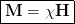#### Types of magnetic materials

The most common materials can be sorted following the way they respond to an external magnetic field in, essentially, three categories : diamagnetic, paramagnetic or ferromagnetic.

Diamagnetic : These materials are characterized by χ < 0, which means that, if they are placed within a certain magnetic field, their dipoles will orientate opposite to it, and will thus be repelled by it. Diamagnetism is actually a property of all materials, but for most of them, it is not the main contribution of their response to magnetic fields. Example mainly diamagnetic materials include water, copper or lead, which display a susceptibility of, typically, about χ ≈ -10-5. Because this value is much smaller than 1, their response will be very weak, which is why water and copper seem to be unaffected by magnetic fields in everyday life. The limiting case of χ = -1 is attained by perfect supraconductors, which have absolutely no magnetic field inside of them (Meissner effect). Diamagnets do not retain an internal magnetic field once the external field has been removed, i.e. they are not permanent magnets.

Paramegnetism : These materials are characterized by χ > 0, which means they will be attracted by magnetic fields. Their susceptibility is generally about χ ≈ 10-5, which again points at a very weak response, non-perceptible in everyday life. An example of such a material is aluminium. Just like diamagnets, paramagnets are not permanent magnets.

Ferromagnetism : These materials are characterized by a susceptibility much greater than 1, and typically a non-linear function of B. They will thus be strongly attracted by magnetic fields, and display a number of properties that are absent from dia- and paramagnets. Among these are their hysteresis behaviour, the presence of Weiss domains and the fact that they can be permanent magnets. Their susceptibility is generally about χ ≈ 100 to 10 000, which denotes a very large response (the magnetic field induced inside the ferromagnet will be much greater than the applied field). A good example of such a material is typically iron and its alloys such as steel.Figure 2.4 — Simplified comparison of permeabilities for: ferromagnets (μf), paramagnets(μp), vacuum (μ0) and diamagnets (μd).
[Image credits: Zureks for Wikipedia]

In a Gauss cannon, we'll want the projectile to respond as much as possible to the applied magnetic field, so that it will be accelerate as efficiently as possible. Using a ferromagnet with a high susceptibility therefore seems to be the best option. We will concern ourselves with the practical matter that is the choice of the optimal material later on (see next page).

## 2.2   Reminders on basic circuits

More basic stuff that will come in handy later, this time on circuit theory. Some of this is already presented on my Zeus Tesla coil page section of reminders, but will be copied here for commodity.

### 2.2.1   Some electrical components

#### Resistor

A resistor is a component that opposes a flowing current. Every conductor has a certain resistance. If one applies a potential difference V at the terminals of a resistor, the current I passing through it is given by :

I = V/R

This formula is know as Ohm’s Law. The SI unit of resistance is the Ohm, noted Ω. One can show that the power P (in J/s) dissipated due to a resistance is equal to

P = VI = RI2

#### Capacitor

A capacitor is a component that can store energy in the form of an electric field. Less abstractly, it is composed in its most basic form of two electrodes separated by a dielectric medium. If there is a potential difference V between those two electrodes, charges will accumulates on those electrodes : a charge Q on the positive electrode and an opposite charge -Q on the negative one. An electrical field therefore arises between them. If both of the electrodes carry the same amount of charge, one can write

Q = CV

where C is the capacity of the capacitor. Its unit is the Farad, noted F. The energy E stored in a capacitor is given by

E = QV/2 = CV2/2

where one can note that the dependence in the charge Q shows that the energy is indeed the energy of the electric field. This corresponds to the amount of work that has to be done to place the charges on the electrodes.

# N.B.

There's a special class of capacitor known as electrolytic capacitors, which can have considerably higher capacitances than standard capacitors, but can only be charged one way (they are said to be "polarised"). This kind of capacitor is usually used in Gauss cannons because of the huge storage capacity they provide. Reverse-charging such a capacitor, however, can lead to catastrophic failure, such as the explosion of the component. While this is an exciting situation to watch, great precautions must be taken to avoid it (obviously), and this will have a considerable impact on the overall cannon behaviour.

#### Inductor

An inductor stores the energy in the form a magnetic field. Every electrical circuit is characterized by a certain inductance. When current flows within a circuit, it generate a magnetic field B that can be calculated from Maxwell-Ampere’s law :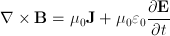where E is the electric field and J is the current density. The auto-inductance of a circuit measures its tendency to oppose a change in current : when the current changes, the flux of magnetic field ΦB that crosses the circuit changes. That leads to the apparition of an "electromotive force"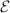that opposes this change. It is given by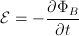The inductance L of a circuit is thus defined as :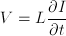where I(t) is the current that flows in the circuit and V the electromotive force (emf) that a change of this current will provoke. The inductance is measured in henrys, noted H.

The energy E stored in an inductor is given by:

E = LI2/2

where the dependence in the current I shows that this energy originates from the magnetic field. It corresponds to the work that has to be done against the emf to establish the current in the circuit.

#### Diode

Although not a "fundamental component", the diode, or p-n junction, plays a very important role in the LC circuit version of the Gauss cannon. Ideally, a diode is a device that allows current to pass in one direction (with zero resistance) while blocking any current that goes in the other direction (infinite resistance). Real-life diodes, however, display a slightly more complicated behaviour, which we will detail now.

Figure 2.5 — Current vs. Voltage plot for an ideal (left) and real-life diode (right).
[Right picture credits: Hldsc for Wikipedia]

We won't have a look at the device's inner workings (which can be found on the related Wikipedia entry, but rather look at the way it works in order to be able to modelise it later. Typically, a diode to which a difference of potential is applied displays three types of behaviour, as indicated in the figure 2.5 above.

Forward mode : The diode is said to be in forward mode when the p-type region is connected to the positive pole. In that configuration, the voltage difference that existed in the so-called depletion zone of the junction is reduced by v. The positive voltage pushes the holes towards the junction, where they meet the electrons pushed away by the negative voltage. The depletion zone henceforth physically shrinks.

If the applied voltage is large enough, i.e. larger than the voltage difference produced by the depletion zone at equilibrium, the depletion zone shrinks so much that it disappears, and the current starts to flow. This gives rise to the green part of the current-voltage plot at figure 2.5.

We thus see that, in order to have a current flowing inside a diode, a small voltage must be overcome. In other words, the presence of a diode in a circuit causes the voltage across the load to drop slightly. This property of diodes is know as the forward voltage drop, and will be very important in our Gauss cannon simulations, which we'll consider later.

Reverse mode : The diode is said to be in reverse mode when the p-type region is connected to the negative pole. Here, the initial voltage difference at the depletion zone is augmented by v. The electrons are attracted towards the positive end while the holes are attracted towards the negative end. The depletion zone becomes larger and no current can flow. This is the blue zone of the plot at figure 2.52.

Breakdown mode : The electrical field within this zone can grow really large (~107 V/m), so that the charge carriers that cross this zone are strongly accelerated. Now if that reverse voltage is larger than a certain threshold value, the carriers can acquire enough kinetic energy to excite electrons from the valence band to the conduction band. A breakdown process, often dubbed "avalanche effect", occurs and a very sharp augmentation of the reverse current is observed. This is depicted on the red zone of the plot at figure 2.5.

#### Silicon-controlled rectifier

A silicon-controlled rectifier (SCR) is another solid-sate device. In a Gauss cannon, it will basically serve as a high-current capacity electrical switch. We will, again, not examine its internal structure here (more details on the Wikipedia page), but rather have a quick look at what the device does.

Figure 2.6 — Left: electrical symbol of a SCR. Right: the "hockey puck"-type SCR in its mounting clamp used on Apollo.

Let us apply a positive voltage difference between the anode and the cathode (the anode having the higher voltage), with no voltage applied to the gate. If this voltage is lower than a certain breakdown voltage (a characteristic of the specific device), then no current will flow. Now, if we apply a small voltage, the junction starts to conduct, and is said to be in forward mode (just like a diode). An important feature of SCRs is that, once in that state, the current will continue to flow even if the gate voltage is no longer maintained. If a negative voltage is applied to the device (i.e. with the anode having the lower voltage), then the junction will typically block any current, regardless of the voltage at the gate. The device is said to be in blocking mode.

SCRs are typically used in high-power applications because of their capacity to handle high voltages and currents. They thus make components of choice to control the massive flow of current we will be having in our Gauss cannon.

### 2.2.2   RC circuit

An RC circuit is composed of a resistor and a capacitor, and possibly of a voltage source. That a kind of circuit is found on the charging section of the Apollo Gauss cannon; its role is to build up the necessary charge into the main capacitor bank to be released upon firing. The specific version of the circuit used on our cannon, and that we're about to analyse, is the series RC circuit, where the capacitor and the resistor are connected in series with a (fixed) voltage source, as shown in figure 2.7. Let's assume that the resistance is of R ohms, the capacitance of C farads, and that the voltage source provides a fixed amount of V0 volts.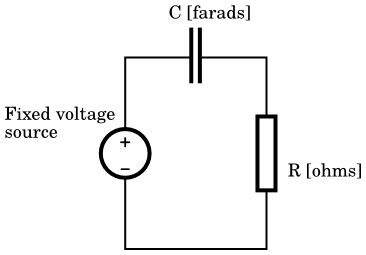Figure 2.7 — Schematic of a series RC circuit driven by a constant voltage source.

We'll now use Kirchoff's laws to find the solution for the voltage V(t) across the capacitor. As our circuit has only one loop, Kirchoff's current law simply tells us the current I(t) is the same everywhere. Kirchoff's voltage laws tells us that :

V0 = Vres(t) + Vcap(t) (2.3)

Now, using the defining equations for the voltage across a resistor (the famous Ohm's law) and across a capacitor that we reminded in the above subsection,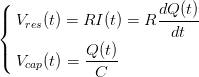we get the following equation :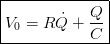(2.4)

where we used Newton's dot notation for time-derivatives. This unhomogeneous differential equation is quite easy to solve. We first look for the so-called "homogeneous solution", where the independent term is zero (V0 = 0 in equation (2.4)), and then look for a "particular solution" (i.e. a preferably easily found solution among all the others), and finally add the two to get our solution. Solving for the homogeneous equation, we thus find: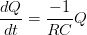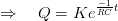(2.5)

which is a decreasing exponential. The coefficient K in front of the exponential is an integration constant (you may check the solution is valid for any fixed value of K) and will be determined afterwards, using the initial condition.

We now look for our particular solution. We can see that "steady-state" version of our circuit, where the time-derivative of Q vanishes is also a solution. Physically, it corresponds to the state where the voltage across the capacitor equals the voltage provided at the source, in other words, when the capacitor is fully charged. The solution we retrieve happens therefore to be, in that case, the defining equation of a capacitor :

Q = V0 C(2.6)

Adding our homogeneous solution given at eq. (2.5), and our particular solution given at eq. (2.6), we find our complete solution :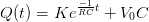(2.7)

We still have to fix the constant K from the initial conditions. As previously said, the way such a RC circuit is used in our Gauss cannon is for charging the main capacitor bank, which are normally empty beforehand. We will thus assume our initial condition are Q(t=0) = 0. When injecting this into eq. (2.7), we find that K = -V0C. Thus our final solution is :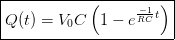(2.8)

In practice, we'll be more interested by knowing the voltage across the capacitors instead of the charge they carry. We simply divide the charge by the capacitance in eq. (2.8) to find the voltage :(2.9)

Although we'll not specifically use it, we can always find the current running into our circuit by time-derivating the charge, given at eq. (2.8) :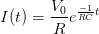The interpretation of these functions is pretty straightforward. The moment we connect our series RC circuit to a voltage generator, the capacitors will accumulate a certain charge in a certain time. Figure 2.8 below plots the charge described in eq. (2.8) versus time.Figure 2.8 — Plot of V(t) for a series RC circuit connected to a fixed voltage generator, with initial condition Q(0) = 0. Values used for the coefficients: V0 = 622, C = 0.029, and R = 200.

We notice that, in the exponential, there's always the -1/RC factor in front of the time t ; this RC constant is called the characteristic time, and one can indeed check that this has the units of a time. It represents the amount of time that the circuit takes to acquire a charge of (1-e)V0C. In more physical terms, it corresponds to the order of magnitude of the time during which the "circuit evolves" (for instance, if tRC, the circuit exhibits a quasi-linear behaviour, while if tRC, the circuit is almost in a steady state). On the plot of fig. 2.8, with the values used for the coefficients, the RC constant is thus 5.8 seconds.

Although we didn't perform the explicit calculation here, one can easily work out what happens in the "opposite" situation, i.e. if there's a charge in the capacitors but no voltage generator. We'd then have an exponential decay of the voltage (and current) from the intial tension, with a characteristic time of RC as well.

### 2.2.3   LC circuit

An LC circuit is formed with a capacitor C and an inductor L, possibly connected in parallel or in series to a voltage generator. The understanding of this circuit is quite important in the Gauss Cannon functioning, as the firing circuit typically contains a massive capacitor bank and inductor, among other things. We'll only analyse the homogeneous LC circuit version (i.e. the "free" circuit, without any voltage generator), as it is the one typically found on Gauss cannons. Note that some cannons use RLC circuits instead of LC circuits, but we'll get to that later.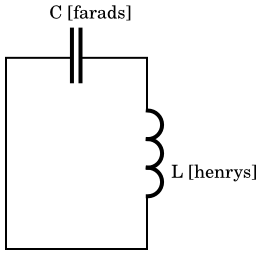Figure 2.9 — Schematic of an homogeneous LC circuit.

Using Kirchoff's law for current, we obtain that the current in the inductor and the current in the capacitor are identical. We now use Kirchoff's law for voltage, which sates that the sum of the voltages across the components along a closed loop is zero, to get the following equation :

0 = VL(t) + VC(t)

For the inductor, we use the expression for V previously found and express the time derivative of current in terms of the charge by I = dQ/dt. We find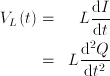Now for the capacitor, we isolate the charge Q in the relation previously found giving the voltage across a capacitor and get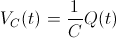Plugging these two results in the equation for Vgen, we obtain (using again Newton's notation) :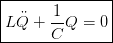(2.10)

This equation describes an (undamped) harmonic oscillator, just like a spring-mass system! The inductor is assimilated to the "mass" of the oscillator : a circuit of great inductance will have a lot of "inertia". The "spring constant" is associated with the inverse of the capacitance 1/C (this is the reason why 1/C is seldom called the "elastance"). The electrical situation described by eq. (2.10) is the following: the capacitor holds a certain charge (or the inductor has a certain current, or both) and we let the system evolute freely. The mechanical analogy with the spring-mass system correspond to an initial state where the spring is tense (or the mass moving, or both) and when no other force interacts.

We find the solution this equation with the characteristic polynomial method. We obtain a sum of complex exponentials, which is of course an oscillating solution as expected.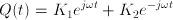where we noted ω = (LC)-1/2 (K1 and K2 are just integration constants). Setting Q(0) ≡ Q0 and Q'(0) = I(0) ≡ 0, which corresponds to the initial condition where the capacitor is fully loaded and no current initially flows in the inductor, we get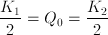We now take the real part of Q(t), but thanks to what we just found, the solution is already real :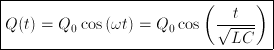We now find the current flows through the inductor by time-derivating the above expression for the charge stored in the capacitor :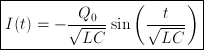The next figures give a good intuition of what actually happens in the circuit. We look at the voltage V(t) between the leads of the capacitor as well as the current I(t) running into the inductor (which is the same in the entire circuit, as previously stated). For convenience, we put all the constants Q0, L, and C to unity.

Figure 2.10 — Left: LC circuits with voltmeter and ammeter. Right: Plot of tension in current versus time.

Step 0:   At the initial instant, the capacitor is fully charged and no current flows. Immediately after, the capacitor begins to discharge: the voltage decreases as a current gradually appears in the circuit.

Step 1:   When the capacitor is totally discharged, the voltages at its leads is zero. If there was no inductance, things would stop right here. However, the inductor opposes this brutal drop of current by generating an electromotive force that will keep the current going. In this way, the current gradually decreases (instead of stopping abruptly) and recharges the capacitor on the way (with an opposite polarity).

Step 2:   The capacitor is fully charged again, with opposite polarity now, and the current has fallen to zero. However the charges stored in the capacitor are willing to neutralize themselves : a current will reappear while the voltage is decreasing again.

Step 3:   The capacitor is "empty" again (zero voltage), but the inductor prevents the current from stopping abruptly. This current will now recharge the capacitor with reversed polarity...

Step 4:   ... and the situation is exactly like it was at the initial instant.

An important feature to note at this point is that the system naturally oscillates at a certain resonant angular speed ω = (LC)-1/2, which is univoquely determined by the inductance and the capacitance of the circuit.

As it is electrolytic capacitors that are typically used in Gauss cannon, we can already remark that we can't use a "pure" LC circuit as firing circuit, because the oscillations will lead to reverse-charging. The way around this is usually to connect a diode in parallel wit the capacitors, and we'll see later how that can drastically alter the cannon's performances.

### 2.2.4   RLC circuit

An RLC circuit is just like an LC circuit with an added resistor. This kind of circuit can take multiple forms. The resistance (Vres(t)=RQ'(t)) dissipates the circuits energy as heat, whatever the direction of the current is, and therefore acts like a friction force. Without a generator, current and voltage will gradually decrease as they oscillate (the circuit is said to be underdamped) or, if the resistance is high enough, no oscillation will take place (overdamped circuit). Since all real-life circuits have internal resistance, the underdamped RLC circuit is actually a much more realistic model than the LC circuit. The limiting case between these two, when the resistance is just high enough to cancel oscillations is called critically damped, and is also of particular interest in the case of Gauss cannon. The reasons for that will be tackled later.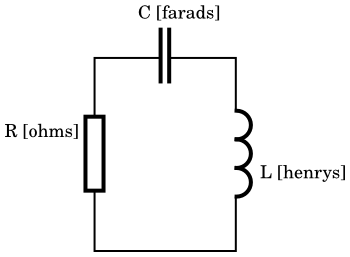Figure 2.11 — Schematic of an homogeneous series RLC circuit.

Again, we will limit our analysis to the version of this circuit that is relevant to the Gauss cannon theory, which is the homogeneous series RLC circuit, where all components are connected in series and no voltage generator is present. Such a circuit is show on fig. 2.11 above.

The equation for current or voltage for a RLC circuit differs from the one for a LC circuit only by the addition of a resistance term. So, by using Kirchoff's laws in the same fashion as in the previous section, we find our equation to be the following :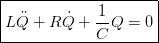(2.11)

We will now solve it for the three aforementioned cases (i.e. underdamped, overdamped and critically damped). We begin by setting the two following quantities, which both have the dimensions of an angular speed and are always positive :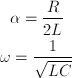(2.12)

Our differential equation thus readsBy using the characteristic polynomial to solve the equation, the three cases we listed will now become clear. Indeed, the roots of the second order polynomial associated with our equation, x2 + 2αx + ω2 are: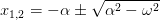Their type (complex or real) and their number are directly influenced by the relative value of α and ω and the following classification naturally arises.

#### Underdamped case : α < ω

In that situation, we have two distinct roots, and our solution will then have the form Q(t) = K1ex1t + K2ex2t, where K1 and K2 are integration constants which will be fixed by the initial conditions. Just as important is the fact that the second term of these roots is purely imaginary. In other words, we then have two complex roots, and our solutions will consist of a sum of complex exponentials, i.e. oscillating solutions.

Let us write the solutions explicitly, with the exponentials already grouped into trigonometric function and initial conditions already computed. We have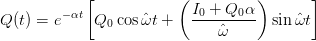(2.13)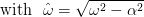(2.14)

where Q0 and I0 respectively represent the charge and the current at the initial moment t = 0. In this free regime, we see that the system carries out oscillations whose amplitude exponentially decrease with time, conversely to the LC circuit where the oscillation amplitude stays constant. We also remark that the natural pulsation of the oscillations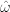(mind your hats) has "shifted" from the LC's one, which was ω = (LC)-1/2. Finally, we notice that at the limit where the attenuation term α goes to zero, we recover the solution for the LC circuit.

In practice, we're interested in the voltage and current flowing into the circuit instead of the sole charge in the capacitors. Also, there will be no initial current in our Gauss cannon, only an initial charge (I0 = 0). Indeed, the capacitor bank will be fully charged, and current will start to flow only after the cannon is fired. Using Q(t) = CV(t), the voltage as a function of time for these intial condition therefore is :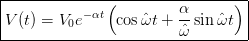(2.15)

While the current, obtained by time-derivating the charge given at eq. (2.13), and still with our aforementioned initial condition I0 = 0, is :(2.16)Figure 2.12 — Plot of voltage (left) and current (right) versus time, as defined by the function given at (2.15) and (2.16), respecively. Values used for the coefficients: C = 0.029, L = 1.97‧10-4, V0 = 450, and R = 0.05.

# N.B.

In the graph above, we used realistic values for our coefficients (capacitance, inductance, etc). In fact, we used the Apollo Gauss cannon's own values, and we will continue to do so in all subsequent plots. We can already see that, in such conditions, the current rises up to more than 3500 amperes.

#### Overdamped case : α > ω

Just as before we have two distinct roots, so the solution will, again, have the form Q(t) = K1ex1t + K2ex2t. But now that the attenuation term α is larger than the oscillation term ω, both these roots are real instead of imaginary, so the exponentials are real as well, and we retreive the behaviour of, typically, exponentially decreasing voltage or current.

The explicit solution, with its initial conditions already computed, takes a very similar form than eq. (2.13), only with hyperbolic functions instead of trigonometric ones :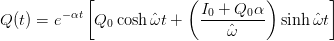(2.17)

with, Q0 and I0 retaining exactly the same meaning. Again, let's calculate the variables of interests from the charge we just calculated, and using intial conditions relevant to Gauss cannon operation (I0 = 0, just as before):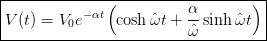(2.18)(2.19)Figure 2.13 — Plot of voltage (left) and current (right) versus time, as defined by the function given at eqs. (2.18) and (2.19), respecively. Values used for the coefficients: C = 0.029, L = 1.97‧10-4, V0 = 450, and R = 1.

#### Critically damped case : α = ω

In that situation, the characteristic polynomial has only one root, -α, and our solution will then have the form Q(t) = (K1 + K2t)ex1t, with x1 being simply equal to -α. The critical damping conditions fixes one of the R,L,C constants. In critically-damped RLC circuit, it will be easier to adjust the resistance, so let's express this constant. We thus have R = 2(L/C)1/2.

Fixing the integration constants from the initial conditions here is very easy. Using, as always, Q(t=0) = Q0 and I(t=0) = Q'(t=0) = I0, one finds K1=Q0 and K2=I0, and our solution for the charge reads :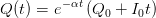(2.20)

As before, let's compute the voltage and the current from our expression for the charge, again with I0 = 0.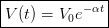(2.21)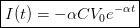(2.22)Figure 2.14 — Plot of voltage (left) and current (right) versus time, as defined by the function given at eqs. (2.21) and (2.22), respectively. Values used for the coefficients: C = 0.029, L = 1.97‧10-4, V0 = 450. R is fixed by the critical damping condition.

## 2.3   Gauss cannon theory of operation

We know have all the theoretical concepts at hand to thoroughly explain the theory of operation of a typical Gauss cannon. We'll start by a very general description of its functioning, then examine more in detail what happens during the firing in itself for our version of the device, i.e. the LC circuit-based Gauss cannon.

### 2.3.1   Description of a firing cycle

The Gauss cannon is actually a pretty simple machine, for its whole cycle of operation can be divided in two fundamental steps : the charging of the capacitor bank, and the discharge into the coil.

#### Charge

In order to have a very massive current to inject into the inductor, we begin by storing a large amount of charges into the main capacitors. This is done by closing the "charge switch", allowing the voltage source to fill up the capacitor through a current-limiting resistor, as indicated on the fig. 2.15 below. We thus see we simply have a RC circuit, just like the one we treated in section 2.2.2, and the voltage across the capacitor will thus build-up with exponentially decreasing speed, as previously shown.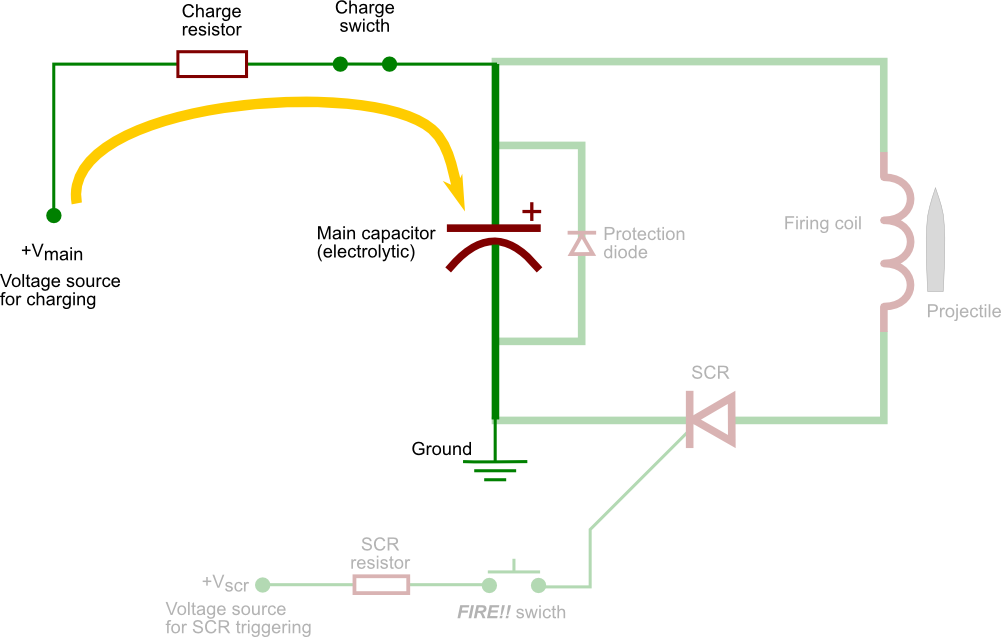Figure 2.15 — Schematic behaviour of the circuit during the charging step, with the relevant section of the circuit highlighted. (The LC version is shown here, but this process is exactly the same on a RLC-based cannon.)

The way one obtains a fixed, DC voltage source is dealt with in the next page, so is the matter of correctly sizing the charging resistor. Once the capacitors are charged up to their full capacity, the charge switch is opened again. The cannon is now ready to be fired.

#### Fire

The firing step simply consists of closing the main, high-current capacity circuit (shown in bold in the schematics). Because of the enormous currents involved, it is not conceivable to use a mechanical switch. Indeed, we are here talking about thousands of amps (we will demonstrate this later on), and any of the common metals used in standard switches would probably melt. There are also obvious safety issues for the operator of the device, who would have to manipulate a switch carrying an extremely dangerous current. For these reasons, we will be using an electrically-controlled switch, and we choose here to use a SCR. As explained earlier, the junction will become conductive when the base is exposed to a comparatively very small current, which is a lot safer to operate.

The firing sequence is initiated when the "fire switch" is closed. This effectively closes the main circuit, allowing current to flow into the firing coil. A magnetic field will thus be created inside the coil (see section 2.1), and, because we've used a ferromagnetic projectile, this magnetic field will induce a magnetisation in it. We are therefore in presence of two magnetic dipoles, and an attractive force between them appears. This is what makes the projectile move. Indeed, the magnetic fields we're creating with the coil are quite intense, and ferromagnetic materials are very sensitive to them. These two factors contribute to the great acceleration the projectile experiences.Figure 2.16 — Simplified depiction of the coil-ferromagnet in our Gauss cannon.

Now, because this current arises from the discharge of a (previously charged) capacitor, we find ourselves in presence of, at first glance, an oscillating circuit LC circuit. At this point, let us pay particular attention to the fact we're using electrolytic capacitors, which can only bear charge one way. We therefore must find a way to cancel the oscillations of our firing circuit, in order not to destroy our capacitor bank after each fire. There are two main options to achieve this goal. Either keep our LC circuit but add a protection diode across the capacitor, or convert it into a critically-damped RLC circuit by adding a dampening resistor in series.

LC version. In this configuration, we add a protection diode across the main capacitor bank, whose role will be to shunt the capacitors as soon as the voltage starts to invert. This way, the capacitors will ideally be spared any catastrophical reverse-charge.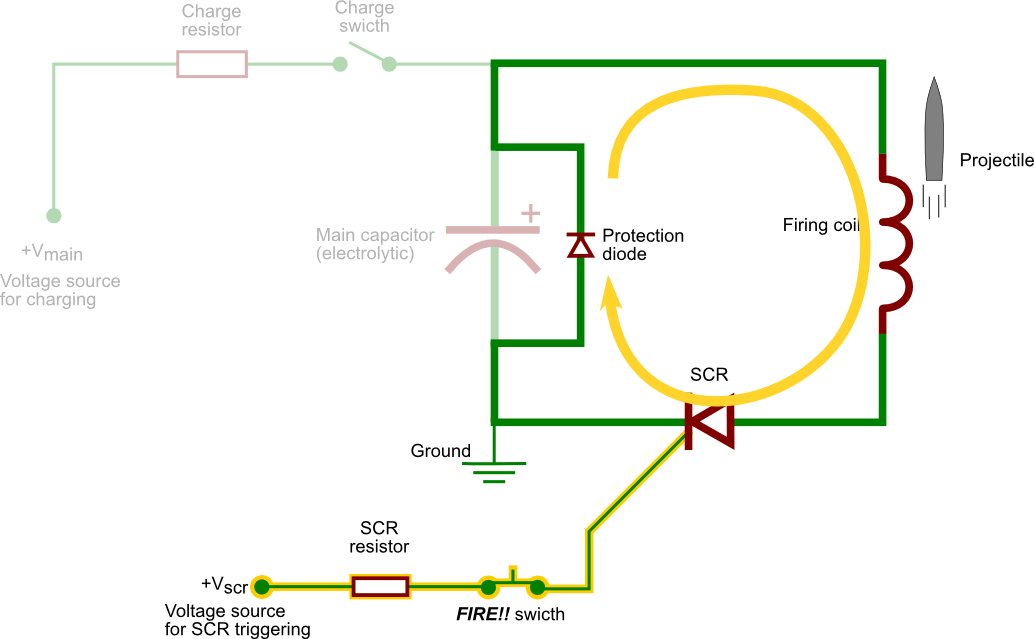Figure 2.17 — Schematic behaviour of the circuit during the firing step in the LC-based Gauss cannon, with the relevant section of the circuit highlighted.

Right after the fire button is pressed, things happen just like they would in a standard LC circuit : voltage goes like a cosine curve while current goes as minus a sine curve, just like shown on section 2.2.3. The diode plays no role until the voltage reaches zero and is on the brink of becoming negative. But after that point, the diode enters in forward mode, effectively redirecting the current around the capacitors, as shown in the fig. 2.17 above, thus sparing the capacitors.

RLC version. Here, a series resistor is added along the firing circuit, with the aim of obtaining a critically-damped RLC circuit. Indeed, we'd like to suppress the oscillations but without losing too much energy in the resistance, and the critical damped configuration offers, per definition, just enough resistance to have a voltage that never goes under zero, as shown in fig 2.14. The capacitors of such a RLC circuit will thus never be reverse-charged.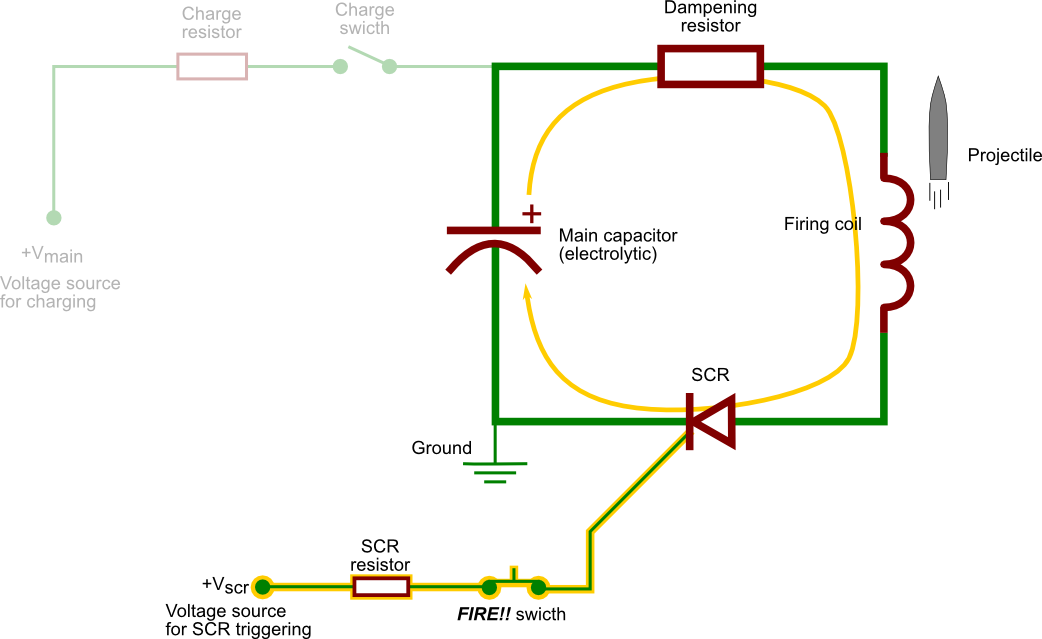Figure 2.18 — Schematic behaviour of the circuit during the firing step in the RLC-based Gauss cannon, with the relevant section of the circuit highlighted.

### 2.3.2   Performance considerations

Before analysing the circuit's behaviour in more detail, let us pause for a moment and examine what are the main factors that must be taken into account in order to have an efficient coilgun.

From our discussion on magnetic fields in section 2.1, it should be clear that, the greater the current we throw into the firing coil, the greater the force on the projectile will be. Although we didn't calculate the force arising between the coil and the magnetized projectile, it is intuitive (and rigorously demonstrated in your Physics 101 course) that the force between two magnets is proportional to the intensity of their respective magnetic fields. Indeed, the magnetic field of the coil is, at first approximation, proportional to the current I (see eq. (2.1)), while the magnetisation of the projectile is, also in a first approximation, proportional to that magnetic field (see eq. (2.2)). Hence, the greater the base current I, the greater the acceleration of the projectile.

Also, an important thing to note is that projectile is attracted towards the middle of the coil (note that we gave no proof of that fact either, but it can easily be understood intuitively when we take into account the fact that we work with dipoles). That means, if there is still current flowing inside the inductor after the projectile passed through its middle, the force acting upon it will tend to suck it back into the coil. This is obviously detrimental to the cannon's performances. Ideally, the current should stop neatly once the projectile has arrived at the middle of the coil. There are circuit designs that can effectively achieve this, such as the "V-switch", but for a basic cannon, we'll simply thrive to have a discharge time as short as possible.

To summarize this discussion, let us just remember that the two most important factors that must be taken into account to have a powerful coilgun are :

• An inductor current as large as possible.
• A discharge time as short as possible.

Now let us see how this can be implemented.

### 2.3.3   Deeper analysis of the fire sequence

The Apollo Gauss cannon was built following the LC with diode paradigm, and this section will describe the behaviour of this circuit in much greater detail. The reasons why I chose the LC version are mostly practical. For one, a critically-damped RLC circuit is quite difficult to realize, especially here, where the critical value of the resistor is very small (due to the fact that we have both a very large capacitance and a rather small inductance, see section 2.2.4). Secondly, a carefully-designed LC cannon can have a higher efficiency than its RLC counterpart.

Our analysis will be done as follows. For one, we will consider the internal resistance of our LC circuit, and thus work with an underdamped RLC circuit, which a much more realistic description of our firing circuit. We will always be able to set this internal resistance to zero when we want to see how an ideal LC circuit would behave. Secondly, we will implement the diode not with a cumbersome step-function, but rather by considering the circuit before and after the diode "takes effect". Before the diode plays a role, we find ourselves in presence of a standard (R)LC circuit. At some moment tswitch during the oscillation, the voltage becomes negative and the diode starts to conduct. Therefore, after that moment, everything will be just like a new branch appeared in the middle of our circuit. The functions for voltage and current will then be obtained by assembling the two previous functions : the standard RLC circuit for t<tswitch and the new circuit for t>tswitch. We will also consider a small resistance along that diode branch. Our approach is summarized in fig 2.19 and by these essential points :

• The main circuit is an underdamped RLC circuit.
• The effect of the diode is to transform the basic RLC circuit into an RLC circuit with an extra branch.
• This switching is done when the voltage across the diode overcomes its forward drop. We'll call this moment tswitch.Figure 2.19 — Our approach of the main firing circuit consists of considering a basic RLC circuit at first, and then a RLC circuit with an extra branch once the diode becomes conductive.

#### Definition of tswitch

Before even laying out our circuit equation, we can already determine the moment tswitch at which we switch from one circuit to the other. This new circuit is supposed to "appear" with the voltage across the diode (which as the same as the voltage across the capacitors) overcomes the forward drop of the diode Vd. For a single diode, this forward drop will typically be only a few volts, and therefore much smaller than the voltages involved in the circuits (several hundred of volts). We will thus consider that Vd≈0. Our moment tswitch will therefore be defined as: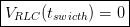Where VRLC(t) is understood as the voltage across the capacitor in our RLC circuit. We will see in a moment that we already computed the right solution for this quantity.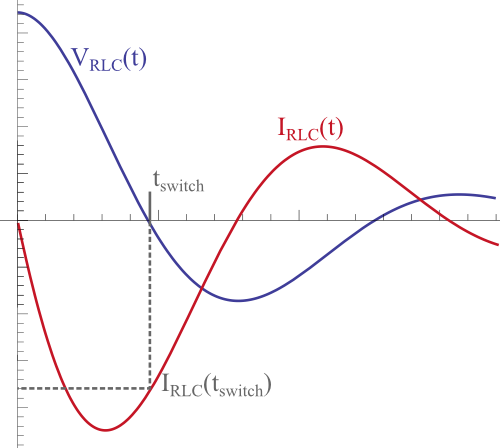Figure 2.20 — Location of tswitch on a typical underdamped RLC circuit time-plot. The value reporter on the y-axis is the current at that moment, which will be useful later.

#### Circuit equations

Let's start by laying out the circuit equation for both before and after the diode takes effect. The t<tswitch situation is our well-know standard RLC circuit, whose circuit equation is given by eq. (2.11), and for which we already computed the solutions for the underdamped case, given by eqs. (2.15) and (2.16). Note that these solutions were obtained with initial conditions corresponding to a fully-charged capacitor and a zero current, which is exactly what we're dealing with in our Gauss cannon. We can thus use these solutions as is, no further work required!

Let us now establish the circuit equation for the t>tswitch case, whose current name conventions are marked on the figure on the right. Using Kirchhoff's law, establishing the system of two equations by summing the contributions on each loop is a straightforward matter. Indeed we obtain :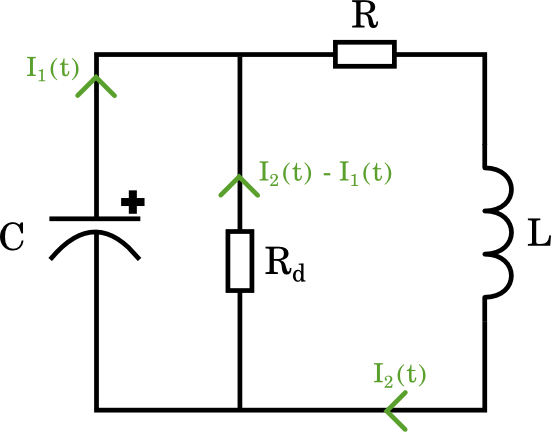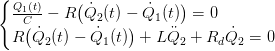Before going any further, let us lay out the initial conditions of this problem. We determined that we'd switch from one circuit to the other when the voltage reaches zero; our initial conditions regarding voltage are thus Q1(0)=0 and Q2(0)=0. This won't be completely true anymore when we will use large racks of diodes to get a forward drop of a several tens of volts, but this choice of conditions does simplify an otherwise extremely complex problem As for the initial current, we will retrieve it by simply calculating the RLC current at the moment the voltage reaches zero. The current is supposed to be the same everywhere before the switching (as per Kirchhoff's law of current), so it seems natural we take the same initial current in both branches. Our initial conditions are thus: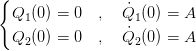(2.23)

with A = IRLC(tswitch) in our standard RLC circuit.

The solution for this equation was found using a mathematical software, and is too cumbersome to be copied here. The interested reader can however find it the resolution on the Mathematica notebook linked on the right. It is now a good time to ask how we should use these Q1(t) and Q2(t) charges (and their respective currents I1(t) and I2(t)), and how do they relate to our solutions for the basic RLC circuit. The answer is very simple: by looking at the schematic, we straightforwardly see we can use Q1(t) to determine the voltage across the capacitors (itself given by Q1(t)/C) and Q2(t) to determine the current into the inductor (given by the time-derivative, dQ2(t)/dt).

Plots of a typical solution for V1(t) and I2(t) are given on fig. 2.21 below.Figure 2.21 — Plot of voltage in the right loop (left) and current in the left loop (right) versus time, as defined by the solutions V1(t) and I2(t) of equations (2.23). Values used for the coefficients: C = 0.029, L = 1.97‧10-4, V0 = 450, R = 0.06, Rd = 0.05.

#### Constructing the piecewise function

We have now everything at hand to construct our complete functions for current and voltage. Indeed, we can write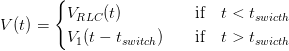(2.24)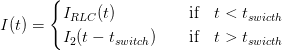(2.25)

Where, as mentioned above, VRLC(t) and IRLC(t) are underdamped solution to the RLC equation, given at eqs. (2.15) and (2.16), V1(t) is the voltage across the capacitors in the left loop of the second circuit and I2(t) is the current flowing into the inductor, in the right loop, both of which are obtained from the solutions to eqs. (2.23).

Plots of such solutions are given on fig. 2.22 below. The values for R, L, C and V0 used are the same as in the functions plotted at figs. 2.12 and 2.20 (recalled in the legend for commodity). Additionally, we obtained tswitch = 4.961⋅10-3 seconds, and IRLC(tswitch) = -2554.9 amps.Figure 2.21 — Plot of voltage (left) and current (right) versus time for the whole firing circuit, using the piecewise functions defined in eqs. (2.24) and (2.25). The grey vertical ruler marks tswitch. Values used for the coefficients: C = 0.029, L = 1.97‧10-4, V0 = 450, R = 0.06, Rd = 0.05.

#### Some solutions of interest

We are now ready to examine a few solutions of interests. This will not only give us precious insight on how the Gauss cannon works but might also clarify the above procedure, as we'll be basically using it with different numerical coefficients.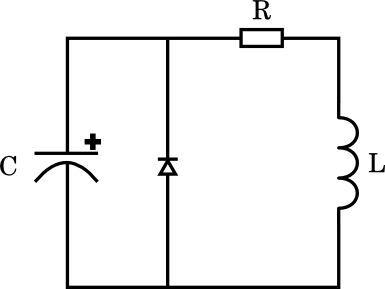Single diode. Let's first consider the situations where there is no resistance along the diode branch, i.e. when Rd ≃ 0. Please note the approximate sign, as, computationally, we can't take this resistance to be strictly zero indeed. The schematic circuit we're dealing with in shown on the right.

We shall begin by neglecting the firing circuit's internal resistance as well (R = 0), which we'll introduce back later on. Before viewing the solution plots, let us pause a moment to examine the situation. Once the voltage drops below zero, the diode becomes conductive and all the current now flows in the right loop. By setting both R and Rd to zero, there's only the inductor left in this loop, so the current that's flowing inside will continue... forever. Indeed, with nothing to dissipate the energy of the system (neglecting the projectile acceleration), the current has no reason to decrease. This is indeed what we see in the following graphs.Figure 2.22 — Plot of voltage (left) and current (right) versus time for the whole firing circuit. Values used for the coefficients: C = 0.029, L = 1.97‧10-4, V0 = 450, R = 0, Rd = 10-7.

This situation is very unrealistic, of course but it already highlights the major conundrum of adding a protection diode, which is the increase in discharge time (here increased to infinity !). When we re-instate the internal resistance of our firing circuit, the current trapped in the second loop will decrease exponentially, and we retrieve a much more physical behaviour. The following plots illustrate this.Figure 2.23 — Plot of voltage (left) and current (right) versus time for the whole firing circuit. Values used for the coefficients: C = 0.029, L = 1.97‧10-4, V0 = 450, R = 0.06, Rd = 10-7.

We see that the current, instead of going on forever as in fig. 2.22, undergoes a exponential decrease, which is what we expect. We can also notice that, in both fig. 2.22 and 2.23, the voltage displays a very sharp stop : once it reaches zero it stays very clearly at that level. This is what we got from setting Rd to zero. Indeed, by doing that, we allow the diode to have a totally unhampered action on the circuit. We'll see in the next paragraph that once we add some resistance in the diode branch, the stop is less brutal (we can already see this in fig. 2.21 where we used Rd = 0.05) because the diode is, in a way, "more hidden from view". One last point of interest is the discharge time. In this case, we see in fig. 2.23 that we can consider t ≃ 0.015 seconds as the point where current is almost extinguished. If we compare to fig 2.21, depicting a pure underdamped RLC circuit, we notice that current crosses the abscissa axis at t ≃ 0.007, which is almost two times less. Keeping in mind that we want our discharge time to be as short as possible, we may consider increasing the Rd resistance in order to shorten our discharge time, which brings us to the next paragraph.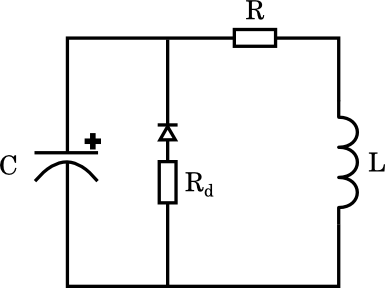Diode with series resistor. We just saw how the protection diode could drastically increase the discharge time. We will now consider increasing the resistance along the diode branch as a means to solve this problem. Indeed, we are in a way making the diode less potent, and in the limiting case where Rd extends to infinity, we retrieve our standard RLC circuit. It is therefore worth analysing what happens as we increase Rd, and see if there's some balanced value that combines both a short discharge time with a limited reverse charge.

We directly consider the underdamped RLC circuit as our firing circuit instead of first looking at the simpler LC circuit, and we will plot our solutions (2.24) and (2.25) just as before, but with various values for Rd.Figure 2.24 — Plot of voltage (left) and current (right) versus time for the whole firing circuit. Values used for the coefficients: C = 0.029, L = 1.97‧10-4, V0 = 450, R = 0.06

We can first notice that, for both current and voltage, the curves look more and more like the curves from a pure RLC as we dial up the Rd resistance. This makes sense, as a very large value for this resistor will lead to a very small current passing through it, thus making the extra branch almost "invisible" to the circuit. Another point of interest lies in the current curve. Starting with Rd ≃ 0, we see that we can effectively reduce the discharge time significantly as we increase the resistance up to about Rd ≃ 0.04 Ohm. Beyond this value, the circuit will start to look like a normal RLC circuit, with current displaying an oscillatory behaviour. While the shortening of the discharge time is very desirable, we must also have a look at the voltages curves too see if the harmful reverse-charging of the capacitors doesn't become too important. For our favourite value of Rd ≃ 0.04 Ohm value, we reach about -60V, which is likely to be acceptable given the short time it will remain that way (about 0.005 seconds).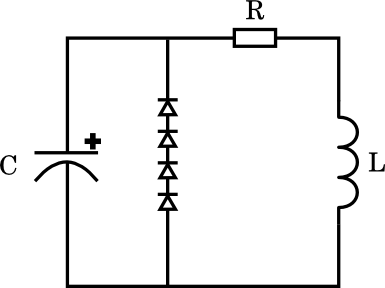Multiple diodes in series. There is one last way we can shorten the discharge time while still protecting our capacitors. By using several diodes in series, we can increase the forward voltage drop of the branch, and thus "delay" the moment at which the protection will kick in. This will, in theory, allow current to flow normally as in a standard RLC circuit for a longer time before introducing the change that will extend the discharge time. We'll see, however, that this strategy alone only works when massive forward drops are used (about -125V for our cannon), which defeats the purpose of protecting the capacitors from reverse-charge in addition to be costly to build.

First of all, the initial conditions given at (2.23) don't hold any more since our forward drop isn't zero any more. Instead, we'll have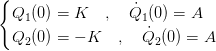(2.26)

Where K is the forward voltage drop of the combined diodes, which is simply the sum of the forward drops of all the diodes in the branch, multiplied by the circuit's capacitance in order to have the units of a charge. Note that K is a negative value. In fact, it turns out the equations of the circuit can't be solved analytically for any value of K. We will thus resort to numerical solving. The graph for the (interpolated) solutions are given below, for various values of K. A quite small series resistance was implemented, Rd = 0.01 Ohms, in order to highlight the contribution made by the forward voltage increase. I did the numerical solving and the plotting with Mathematica script given on the right.Figure 2.25 — Plot of voltage (left) and current (right) versus time for the whole firing circuit. Values used for the coefficients: C = 0.029, L = 1.97‧10-4, V0 = 450, R = 0.06, Rd = 0.01

We first notice that, to achieve our initial goal of reducing the discharge time, we have to use a very large forward drop. Indeed, the current plot shows us that significant reduction only occurs at K ≃ -125V. In practice, this will be quite difficult to achieve, given that most diodes have forward drops of only a few volts. Moreover, this will allow for the capacitors to bear against 125V of reverse voltage, as show in the voltage plot, which is quite a large value for capacitors designed to hold 450V of positive charge. Now, given that the duration of this reverse-charge situation is very short (only about 0.003 seconds), it might be possible that the capacitors survive. I haven't tested whether or not this is possible, but it is certainly a dangerous bet, and is also likely to reduce the capacitors' lifetime. However, we can see that the current discharge is reduced even more than the in the previous circuit paradigm.

#### Conclusions on capacitor protection

We have seen how the protection of the capacitors leads to an increase in discharge time and we presented several ways to mitigate this fact. In all approaches, however, there is always a trade-off between discharge time and reverse voltage. Often, the most cost-effective solution will be to add a carefully-sized series resistor to the capacitor's branch, as we'll see in the next page.

That's it for the theory of operation ! We have reviewed the physical phenomena that are behind the acceleration of the projectile inside the cannon, and given a detailed analysis of the firing circuit equation, following a model that gave coherent answers. The next page will deal with the actual construction of the coilgun, and will also discuss some "non-essential" theory chapters, such as magnetic reluctance and Foucault currents from a practical point of view.